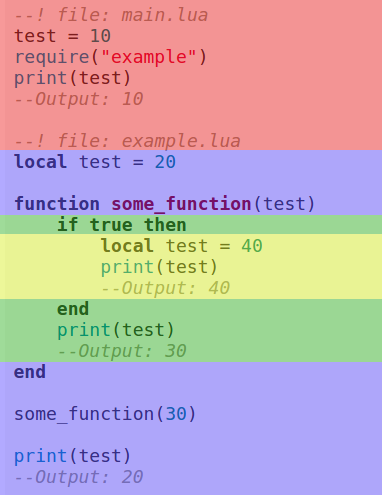Learn how to program games with the LÖVE framework

# Chapter 9 - Multiple files and scope

## Multiple files

With multiple files our code will look more organized and easier to navigate. Create a new file called `example.lua`. Make sure it's in the same folder as a new and empty `main.lua`.

Inside this file, create a variable. I will put `--! file:` at the top of the codeblock to make clear in what file you have to put the code. This is only for this tutorial, it has no use (it's only commentary after all) and you don't need to copy it. If a codeblock doesn't have this in future tutorials, it's either in main.lua, or the previous mentioned file.

``````--! file: example.lua
test = 20``````

Now in `main.lua`, put `print(test)`. When you run the game, you'll see that `test` equals `nil`. This is because we have to load the file first. We do this with `require`, by passing the filename as string as first argument.

``````--! file: main.lua
--Leave out the .lua
-- No need for love.load or whatever
require("example")
print(test)``````

We don't add the `".lua"` in the filename, because Lua does this for us.

You can also put the file in a subdirectory, but in that case make sure to include the whole path.

``````--With require we use . instead of /
require("path.to.example")``````

Now when you print `test`, after we loaded `example.lua`, you should see it says 20.

`test` in this case is what we call a global variable. It's a variable that we can use everywhere in our project. The opposite of a global variable, is a local variable. You create a local variable by writing `local` in front of the variable name.

``````--! file: example.lua
local test = 20``````

Run the game to see test is now `nil` again. This is because of its scope.

## Scope

Local variables are limited to their scope. In the case of `test`, the scope is the file `example.lua`. This means that `test` can be used everywhere inside that file, but not in other files.

If we were to create a local variable inside a block, like a function, if-statement or for-loop, then that would be the variable's scope.

``````--! file: example.lua
if true then
local test = 20
end

print(test)
--Output: nil``````

`test` is `nil`, because we print it outside of its scope.

Parameters of functions are like local variables. Only existing inside the function.

To really understand how scope works, take a look at the following code:

``````--! file: main.lua
test = 10
require("example")
print(test)
--Output: 10``````
``````--! file: example.lua
local test = 20

function some_function(test)
if true then
local test = 40
print(test)
--Output: 40
end
print(test)
--Output: 30
end

some_function(30)

print(test)
--Output: 20``````

If you run the game, it should print: 40, 30, 20, 10. Let's take a look at this code step by step.

First we create the variable `test` in `main.lua`, but before we print it we require `example.lua`.

In `example.lua` we create a local variable `test`, which does not affect the global variable in `main.lua`. Meaning that the value we give to this local variable we create is not given to the global variable.

We create a function called `some_function(test)` and then call that function.

Inside that function the parameter `test` does not affect the local variable we created earlier.

Inside the if-statement we create another local variable called `test`, which does not affect the parameter `test`.

The first print is inside the if-statement, and it's 40. After the if-statement we print `test` again, and now it's 30, which is what we passed as argument. The parameter `test` was not affected by the `test` inside the if-statement. Inside the if-statement the local variable took priority over the parameter.

Outside of the function we also print `test`. This time it's 20. The `test` created at the start of `example.lua` was not affected by the `test` inside the function.

And finally we print `test` in `main.lua`, and it's 10. The global variable was not affected by the local variables inside `example.lua`.

I made a visualization of the scope of each `test` to make it even more clear:When creating a local variable, you don't have to assign a value right away.

``````local test
test = 20``````

## Returning a value

Like functions, files can return a value. If we put `return 10` at the end of `example.lua`, and inside `main.lua` we do `print(require("example"))`, you will see that it prints 10.

## Summary

With `require` we can load other lua-files. When you create a variable you can use it in all files. Unless you create a local variable, which is limited to its scope. Local variables do not affect variables with the same name outside of their scope.

Do you need help or do you see a mistake?